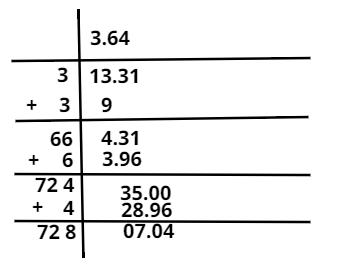Courses
Courses for Kids
Free study material
Free LIVE classes
MoreLIVE
Join Vedantu’s FREE Mastercalss

# Find the square root of $13.31$ using a long division method.Verified
365.4k+ views
Hint : Drawing table will make it easier.

Step I: Group the digits in pairs, starting with the digit in the units place. Each pair and the remaining digit (if any) is called a period.

Step II: Think of the largest number whose square is equal to or just less than the first period. Take this number as the divisor and also as the quotient.

Step III: Subtract the product of the divisor and the quotient from the first period and bring down the next period to the right of the remainder. This becomes the new dividend.
Step IV: Now, the new divisor is obtained by taking two times the quotient and annexing with it a suitable digit which is also taken as the next digit of the quotient, chosen in such a way that the product of the new divisor and this digit is equal to or just less than the new dividend.

Step V: Repeat steps (2), (3) and (4) till all the periods have been taken up. Now, the quotient so obtained is the required square root of the given number.Note :- In this method of finding the square root , in addition to the above steps we say that take care of signs and where to place the number. Then follow the above steps carefully. Since everything regarding this method is mentioned above.

Last updated date: 29th Sep 2023
Total views: 365.4k
Views today: 6.65k